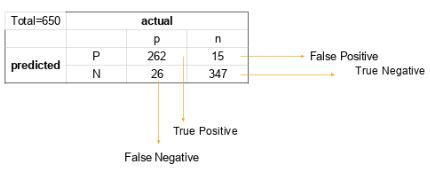Write the equation and calculate the precision and recall rate.

Q:
Write the equation and calculate the precision and recall rate.

Consider the same confusion matrix used in the previous question.Precision = (True positive) / (True Positive + False Positive)

= 262 / 277

= 0.94

Recall Rate = (True Positive) / (Total Positive + False Negative)

= 262 / 288

= 0.90

+1 vote